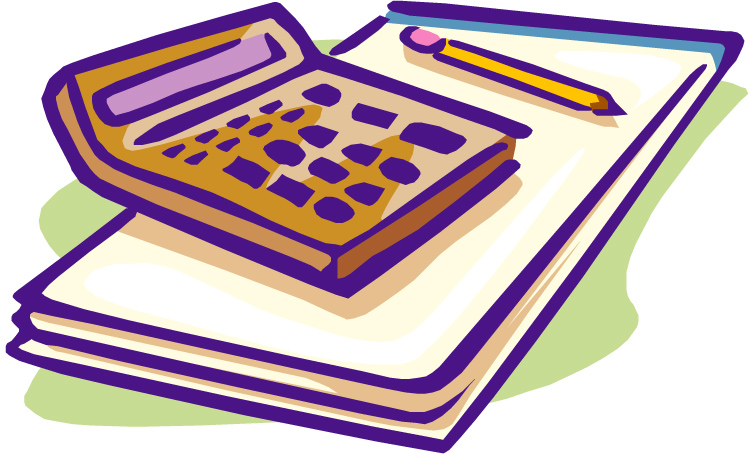Knowledge is Power

Whenever you're here at TarotSchool.com, you can use our handy online Birth Card Calculator to determine anyone's Birth Cards. Just enter their date of birth and click the "Calculate" button — it's easy!

But by learning how to do the calculations yourself, you can figure them out anytime whether you're near a computer or not. This is especially useful when you're conducting in-person or phone readings. Knowing a querent's Birth Cards can add a lot to the reading.

Understanding how to calculate Birth Cards can be a big hit at parties and other social gatherings, too!

When working with The Tarot School system of Birth Cards, please use the method described here even though you may have seen it done differently elsewhere. We have found that the difference between this method and others produces slightly different results, and that most people identify more closely with the results we get by using the formula below.

The Basic Formula:

You can determine your Birth Cards by doing a numerological reduction of your birth date in the following manner: MM + DD + 19* + YY.

* For people born from January 1, 2000 – December 31, 2099, the constant will be 20 instead of 19.

For example, let's say that your birthday is February 21, 1950. Add the numbers like this:

02
21
19
50

92

Then add 9 + 2 = 11.

Key 11 is Justice, the first Birth Card for this date. 11 reduces to 2 (The High Priestess), which is the second Birth Card.

Three-Digit Sums:

Here's how to handle a birth date that adds up to 3 digits. The first two digits of the three are considered as one number, which is added to the third digit. Let's look at August 20, 1969, for example. The formula would be 08 + 20 + 19 + 69 = 116.

Or you can look at it this way:

08
20
19
69

116

Then add 11 + 6 = 17.

11
06

17

Key 17 is The Star, the first Birth Card for this date. 17 reduces to 8 (Strength) which is the second Birth Card. If the three numbers are 103, for example, add 10 + 3 = 13 (Death) = 4 (Emperor).

Single-Digit Sums:

Almost everyone should have 2 birth cards. One of the cards will have a single digit and the other a double digit. If your birth date sum reduces to a single digit (e.g. 90 = 9 + 0 = 9) its pair is implied, in this case, 18 (The Moon) = 1 + 8 = 9 (Hermit). See the table below for a complete list of Birth Card Pairs.

The Exception to the Rule:

The one exception is if your birth date sum = 19. In that case, you will have 3 Birth Cards: The Sun (19), The Wheel of Fortune (10) and The Magician (1).

Clarification:

Confusion about calculating Birth Cards is very common. Even though the math is simple, the concept can be difficult to grasp. In case we've lost you, consider the following:

If a birthday adds up to a digit plus a zero, it reduces immediately to a single digit. But each single digit is paired with a double digit between 10 and 21, from which it is derived. The numbers 1 through 21, of course, are the complete universe of positive numbers that make up the Major Arcana. So all Birth Card pairs must be a combination of two of these numbers. 9, for instance, can only be arrived at, within the numbers of the Major Arcana, from the number 18 (1+8=9).

If you begin with a single digit and you search for the double digit that is its pair, you will find that there is only one possibility, with a single exception. If your birth date adds up to 30, it reduces to 3. Three can be arrived at by a reduction of either 12 (Hanged Man) or 21 (World), but we have found that where this choice exists, 12 works best.

Table of Birth Card Pairs:

 18 reduces to 9 (1+8) Moon / Hermit 17 reduces to 8 (1+7) Star / Strength 16 reduces to 7 (1+6) Tower / Chariot 15 reduces to 6 (1+5) Devil / Lovers 14 reduces to 5 (1+4) Temperance / Hierophant 13 reduces to 4 (1+3) Death / Emperor 12 reduces to 3 (1+2) Hanged Man / Empress 11 reduces to 2 (1+1) Justice / High Priestess 10 reduces to 1 (1+0) Wheel of Fortune / Magician

Again, there is one exception where you will have three Birth Cards. If the birth date sum = 19, it will reduce first to (19), then to (10), and finally (1). Sun / Wheel / Magician

 20 reduces to 2 (2+0) Judgement / High Priestess 21 reduces to 3 (2+1) World / Empress

In most decks, The Fool is either un-numbered, or is assigned the digit 0. Since no sum of positive numbers ever equals 0, we do not consider The Fool as part of a Birth Card pair. Likewise, we do not accept the sometimes-used convention of assigning the number 22 to The Fool since there is no such trump. However, we do consider The Fool to be the universal birthright of us all.Get Tarot School Birth Card icons on mugs, t-shirts and more!

BACK

Top

Birth Card icons by Robert M. Place. Copyright The Tarot School.
Card images are from the Universal Waite Tarot Deck, Copyright US Games Inc.